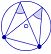Go to contentAB is a chord of the circle. C and D are points on the circumference. Use your mouse to change the size of the circle,or the positions of the centre, points A, B, C or D. Observe the angles at C and D.

However you alter the diagram, the angles at C and D will remain identical to each other. Thus...

The angles in the same segment are equalJSXGraph is a cross-browser library for interactive geometry, function plotting, charting, and data visualization in a web browser. It is implemented completely in JavaScript, does not rely on any other library. It uses SVG and VML and is fully HTML5 compliant.

## Glossary

### chord

A straight line joining two points on a circle or other curve.

### circle

a conic curve with equation (x-a)²+(y-b)²=r²

### function

A rule that connects one value in one set with one and only one value in another set.

Full Glossary List

## This question appears in the following syllabi:

SyllabusModuleSectionTopicExam Year
AQA AS Maths 2017Pure MathsStraight Lines and CirclesCircle Theorems-
AQA AS/A2 Maths 2017Pure MathsStraight Lines and CirclesCircle Theorems-
AQA GCSE (9-1) Higher (UK)G: Properties and ConstructionsG10: Circle TheoremsCircle Theorems-
CBSE IX (India)GeometryCirclesTheorems-
CBSE X (India)GeometryCirclesLengths of tangents from external point are equal-
CIE IGCSE (9-1) Maths (0626 UK)3 GeometryB3.7 Calculating Unknown AnglesCircle Theorems-
Edexcel AS Maths 2017Pure MathsCirclesCircle Theorems-
Edexcel AS/A2 Maths 2017Pure MathsCirclesCircle Theorems-
Edexcel GCSE (9-1) Higher (UK)G: Properties and ConstructionsG10: Circle TheoremsCircle Theorems-
GCSE Higher (UK)GeometryCirclesTheorems-
OCR AS Maths 2017Pure MathsLines and CirclesCircle Theorems-
OCR GCSE (9-1) Higher (UK)8: Basic Geometry8.05: Circle TheoremsCircle Theorems-
OCR MEI AS Maths 2017Pure MathsLines and CirclesCircle Theorems-
Universal (all site questions)CCirclesTheorems-